Common Lisp the Language, 2nd EditionNext: Branch CutsPrincipal Up: Irrational and Transcendental Previous: Exponential and Logarithmic

## 12.5.2. Trigonometric and Related Functions

Some of the functions in this section, such as abs and signum, are apparently unrelated to trigonometric functions when considered as functions of real numbers only. The way in which they are extended to operate on complex numbers makes the trigonometric connection clear.

[Function]
abs number

Returns the absolute value of the argument. For a non-complex number x,

```(abs x) == (if (minusp x) (- x) x)
```

and the result is always of the same type as the argument.

For a complex number z, the absolute value may be computed as

```(sqrt (+ (expt (realpart z) 2) (expt (imagpart z) 2)))
```

Implementation note: The careful implementor will not use this formula directly for all complex numbers but will instead handle very large or very small components specially to avoid intermediate overflow or underflow.

For example:

```(abs #c(3.0 -4.0)) => 5.0
```

The result of (abs #c(3 4)) may be either 5 or 5.0, depending on the implementation.

[Function]
phase number

The phase of a number is the angle part of its polar representation as a complex number. That is,

```(phase z) == (atan (imagpart z) (realpart z))
```The result is in radians, in the range -pi (exclusive) to +pi (inclusive). The phase of a positive non-complex number is zero; that of a negative non-complex number is pi. The phase of zero is arbitrarily defined to be zero.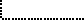X3J13 voted in January 1989 (IEEE-ATAN-BRANCH-CUT)   to specify certain floating-point behavior when minus zero is supported; phase is still defined in terms of atan as above, but thanks to a change in atan the range of phase becomes -pi inclusive to +pi inclusive. The value - results from an argument whose real part is negative and whose imaginary part is minus zero. The phase function therefore has a branch cut along the negative real axis. The phase of +0+0i is +0, of +0-0i is -0, of -0+0i is +pi, and of -0-0i is -pi.If the argument is a complex floating-point number, the result is a floating-point number of the same type as the components of the argument. If the argument is a floating-point number, the result is a floating-point number of the same type. If the argument is a rational number or complex rational number, the result is a single-format floating-point number.

[Function]
signum number

By definition,

```(signum x) == (if (zerop x) x (/ x (abs x)))
```

For a rational number, signum will return one of -1, 0, or 1 according to whether the number is negative, zero, or positive. For a floating-point number, the result will be a floating-point number of the same format whose value is -1, 0, or 1. For a complex number z, (signum z) is a complex number of the same phase but with unit magnitude, unless z is a complex zero, in which case the result is z. For example:

```(signum 0) => 0
(signum -3.7L5) => -1.0L0
(signum 4/5) => 1
(signum #C(7.5 10.0)) => #C(0.6 0.8)
(signum #C(0.0 -14.7)) => #C(0.0 -1.0)
```

For non-complex rational numbers, signum is a rational function, but it may be irrational for complex arguments.

[Function]
sin radians
cos radians
tan radians

sin returns the sine of the argument, cos the cosine, and tan the tangent. The argument is in radians. The argument may be complex.

[Function]
cis radians

This computes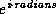. The name cis means ``cos + i sin,'' because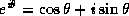. The argument is in radians and may be any non-complex number. The result is a complex number whose real part is the cosine of the argument and whose imaginary part is the sine. Put another way, the result is a complex number whose phase is the equal to the argument (mod 2) and whose magnitude is unity.

Implementation note: Often it is cheaper to calculate the sine and cosine of a single angle together than to perform two disjoint calculations.

[Function]
asin number
acos number

asin returns the arc sine of the argument, and acos the arc cosine. The result is in radians. The argument may be complex.

The arc sine and arc cosine functions may be defined mathematically for an argument z as follows:

```
Arc sine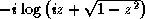Arc cosine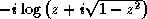```
Note that the result of asin or acos may be complex even if the argument is not complex; this occurs when the absolute value of the argument is greater than 1.Kahan  suggests for acos the defining formula

```
Arc cosine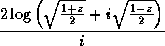```
or even the much simpler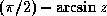. Both equations are mathematically equivalent to the formula shown above.Implementation note: These formulae are mathematically correct, assuming completely accurate computation. They may be terrible methods for floating-point computation. Implementors should consult a good text on numerical analysis. The formulae given above are not necessarily the simplest ones for real-valued computations, either; they are chosen to define the branch cuts in desirable ways for the complex case.

[Function]
atan y &optional x

An arc tangent is calculated and the result is returned in radians.

With two arguments y and x, neither argument may be complex. The result is the arc tangent of the quantity y/x. The signs of y and x are used to derive quadrant information; moreover, x may be zero provided y is not zero. The value of atan is always between -pi (exclusive) and +pi (inclusive). The following table details various special cases.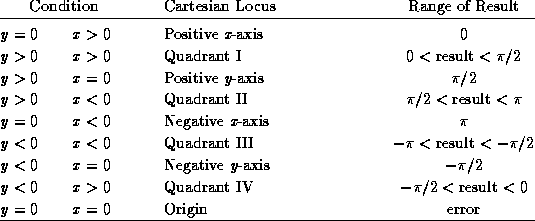X3J13 voted in January 1989 (IEEE-ATAN-BRANCH-CUT)   to specify certain floating-point behavior when minus zero is supported. When there is a minus zero, the preceding table must be modified slightly: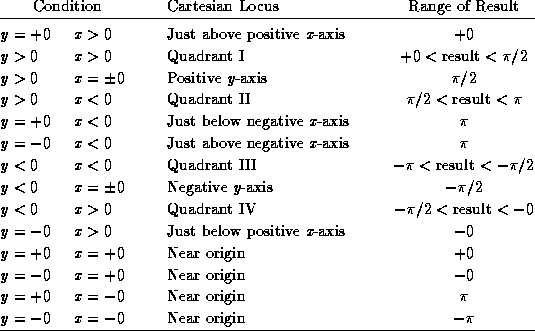Note that the case y=0,x=0 is an error in the absence of minus zero, but the four cases y=0,x=0 are defined in the presence of minus zero.With only one argument y, the argument may be complex. The result is the arc tangent of y, which may be defined by the following formula:

```Arc tangent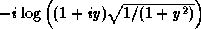```Implementation note: This formula is mathematically correct, assuming completely accurate computation. It may be a terrible method for floating-point computation. Implementors should consult a good text on numerical analysis. The formula given above is not necessarily the simplest one for real-valued computations, either; it is chosen to define the branch cuts in desirable ways for the complex case.X3J13 voted in January 1989 (COMPLEX-ATAN-BRANCH-CUT)   to replace the preceding formula with the formula

```                        log(1+iy) - log(1-iy)
Arc tangent		---------------------
2i
```
This change alters the direction of continuity for the branch cuts, which alters the result returned by atan only for arguments on the imaginary axis that are of magnitude greater than 1. See section 12.5.3 for further details.For a non-complex argument y, the result is non-complex and lies between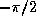and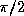(both exclusive).

Compatibility note: MacLisp has a function called atan whose range is from 0 to 2. Almost every other programming language (ANSI Fortran, IBM PL/1, Interlisp) has a two-argument arc tangent function with range -pi to +pi. Lisp Machine Lisp provides two two-argument arc tangent functions, atan (compatible with MacLisp) and atan2 (compatible with all others).

Common Lisp makes two-argument atan the standard one with range -pi to +pi. Observe that this makes the one-argument and two-argument versions of atan compatible in the sense that the branch cuts do not fall in different places. The Interlisp one-argument function arctan has a range from 0 to pi, while nearly every other programming language provides the rangetofor one-argument arc tangent! Nevertheless, since Interlisp uses the standard two-argument version of arc tangent, its branch cuts are inconsistent anyway.

[Constant]
pi

This global variable has as its value the best possible approximation to pi in long floating-point format. For example:

```(defun sind (x)     ;The argument is in degrees
(sin (* x (/ (float pi x) 180))))
```

An approximation to pi in some other precision can be obtained by writing (float pi x), where x is a floating-point number of the desired precision, or by writing (coerce pi type), where type is the name of the desired type, such as short-float.

[Function]
sinh number
cosh number
tanh number
asinh number
acosh number
atanh numberThese functions compute the hyperbolic sine, cosine, tangent, arc sine, arc cosine, and arc tangent functions, which are mathematically defined for an argument z as follows:

```Hyperbolic sine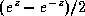Hyperbolic cosine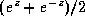Hyperbolic tangent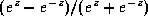Hyperbolic arc sine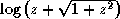Hyperbolic arc cosine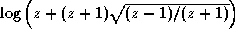Hyperbolic arc tangent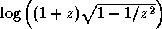WRONG!
```WARNING! The formula shown above for hyperbolic arc tangent is incorrect. It is not a matter of incorrect branch cuts; it simply does not compute anything like a hyperbolic arc tangent. This unfortunate error in the first edition was the result of mistranscribing a (correct) APL formula from Penfield's paper . The formula should have been transcribed as

```
Hyperbolic arc tangent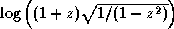```
A proposal was submitted to X3J13 in September 1989 to replace the formulae for acosh and atanh. See section 12.5.3 for further discussion.Note that the result of acosh may be complex even if the argument is not complex; this occurs when the argument is less than 1. Also, the result of atanh may be complex even if the argument is not complex; this occurs when the absolute value of the argument is greater than 1.

Implementation note: These formulae are mathematically correct, assuming completely accurate computation. They may be terrible methods for floating-point computation. Implementors should consult a good text on numerical analysis. The formulae given above are not necessarily the simplest ones for real-valued computations, either; they are chosen to define the branch cuts in desirable ways for the complex case.Next: Branch CutsPrincipal Up: Irrational and Transcendental Previous: Exponential and Logarithmic

AI.Repository@cs.cmu.edu